False position method

(diff) ← Older revision | Latest revision (diff) | Newer revision → (diff)

Definition

False position method is a root-finding algorithm that is qualitative similar to the bisection method in that it uses nested intervals based on opposite signs at the endpoints to converge to a root, but is computationally based on the secant method.

Unless otherwise specified, the function will be denoted$f$.

Initial exploratory phase

The exploratory phase of the false position method involves finding a pair of input values at which the function has opposite signs. This could be done by running the usual secant method and evaluating at each stage until we get to opposite signs, or by some other means. Once we have found two points in the domain at which the function value has opposite signs, we are ready to begin the false position method proper.

In the point estimate version of the iterative step, we will label these two initial guesses as$x_0$ and$x_1$ (so that$f(x_0)$ and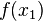$f(x_1)$ have opposite sign). In the nested interval version, we will label the smaller of them as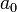$a_0$ and the larger as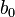$b_0$.

Iterative step

Point estimate version

At stage$n$, for$n \ge 2$, we find the largest$k$ for which$f(x_k)$ has sign opposite to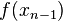$f(x_{n-1})$. We then define:$x_n := \frac{x_kf(x_{n-1}) - x_{n-1}f(x_k)}{f(x_{n-1}) - f(x_k)}$

More details are below.

Prior knowledge: Prior to beginning stage$n$:

• We have a previous set of guesses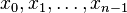$x_0, x_1, \dots, x_{n-1}$.
• We know that$f(x_0)$ and$f(x_1)$ have opposite sign to each other (this is the initial condition). Therefore, among the values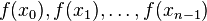$f(x_0), f(x_1), \dots, f(x_{n-1})$, we know that there are both positive and negative values.

Iterative step:

• We find the largest$k$ such that$f(x_k)$ and$f(x_{n-1})$ have opposite sign.
• We compute:$x_n := \frac{x_kf(x_{n-1}) - x_{n-1}f(x_k)}{f(x_{n-1}) - f(x_k)}$

Nested interval version

We start with an initial interval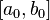$[a_0,b_0]$ (here$a_0$ is the smaller of$x_0$ and$x_1$ from the original setup, and$b_0$ is the larger of them).

At stage$n$ for$n$ a positive integer

Prior knowledge:

•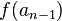$f(a_{n-1})$ and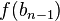$f(b_{n-1})$ are both numerically distinguishable from zero (so they have defined signs, positive or negative) and they have opposite signs.
• Combining that with the fact that$f$ is a continuous function, the intermediate value theorem tells us that$f$ has a root on$[a_{n-1},b_{n-1}]$.

Iterative step:

• Compute:$x_n := \frac{b_{n-1}f(a_{n-1}) - a_{n-1}f(b_{n-1})}{f(a_{n-1}) - f(b_{n-1})}$

Note that since$f(a_{n-1})$ and$f(b_{n-1})$ have opposite signs, this is a convex combination, and therefore$x_n \in [a_{n-1},b_{n-1}]$.

• In the case that$f(x_n) = 0$ (or is numerically indistinguishable from zero), we declare that as the root and terminate the algorithm.
• In the case that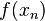$f(x_n)$ has opposite sign to$f(a_{n-1})$ (and therefore the same sign as$f(b_{n-1})$), the new interval$[a_n,b_n] = [a_{n-1},x_n]$. Explicitly,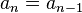$a_n = a_{n-1}$ and$b_n = x_n$.
• In the case that$f(x_n)$ has opposite sign to$f(b_{n-1})$ (and therefore the same sign as$f(a_{n-1})$), the new interval$[a_n,b_n] = [x,b_{n-1}]$. Explicitly,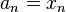$a_n = x_n$ and$b_n = b_{n-1}$.

The values of$x_n$ as obtained here are the same as the values of$x_n$ in the preceding description. The main advantage of this description is that we are storing the intervals as we construct them rather than merely the point estimates, so that some aspects of how the procedure works are more transparent.

Convergence rate

Convergence for linear functions

In the case that$f$ is linear, we reach the root in one iteration after we have found values where the function has opposite signs. In fact, even if we applied the secant method directly, we would terminate in one step.

Convergence for twice differentiable functions

Fill this in later